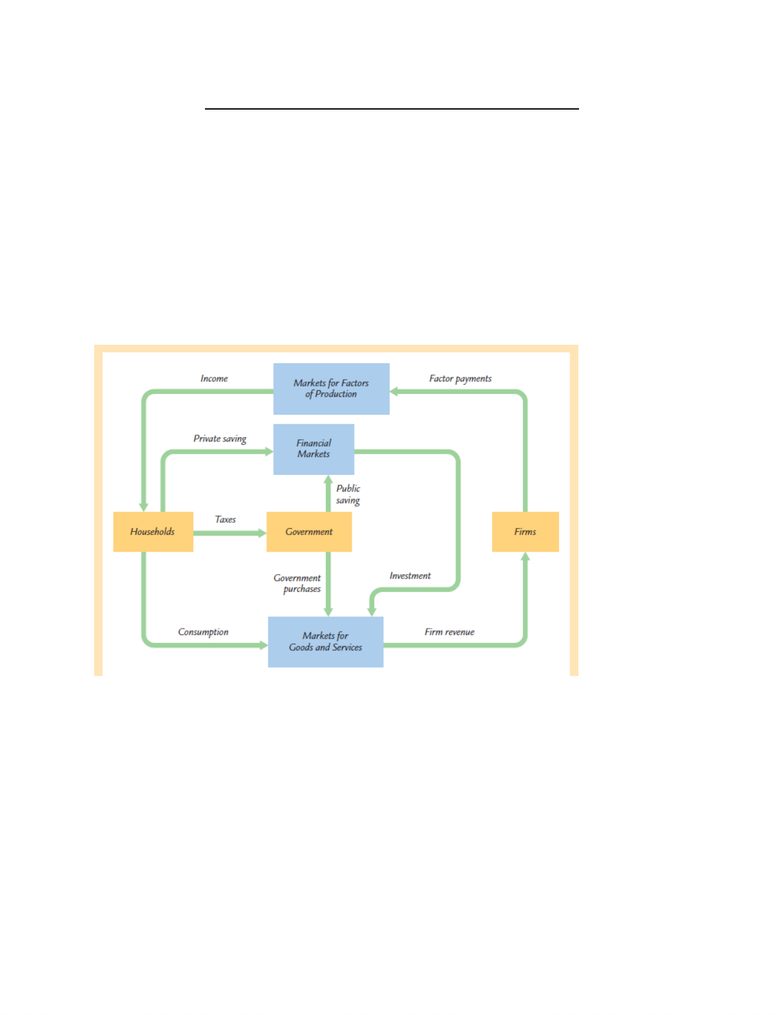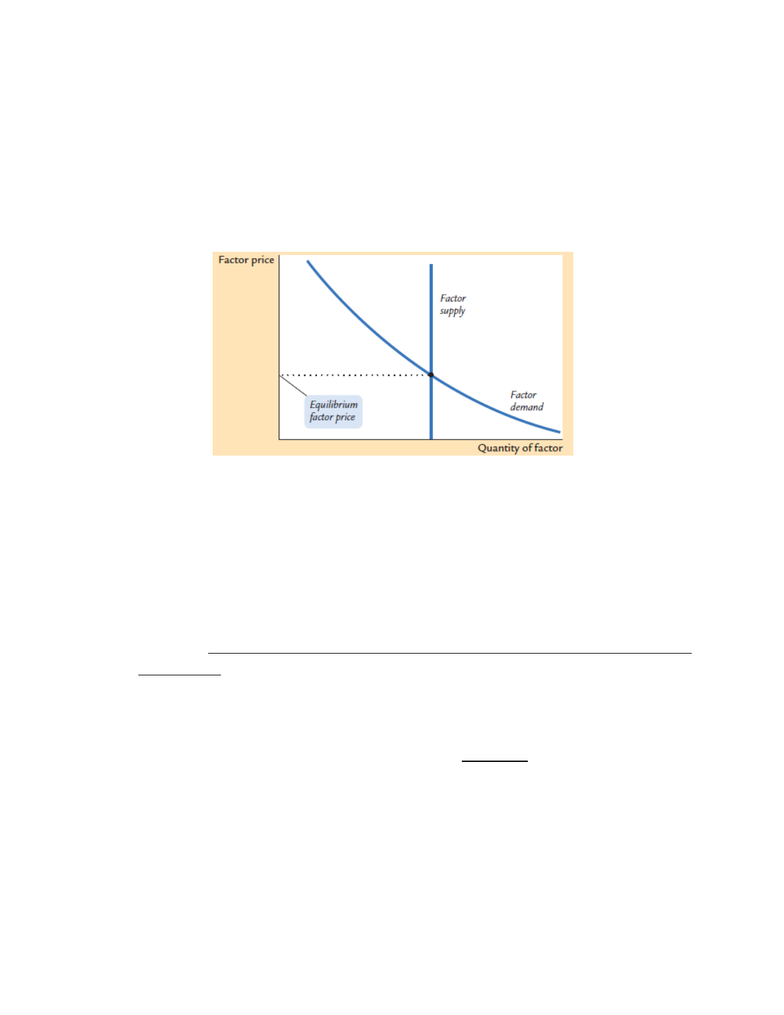Study Guides (390,000)
CA (150,000)
ECON (4)
Final

# ECON202 Study Guide - Final Guide: Aggregate Supply, Demand Curve, Consumption Function

Department
Economics
Course Code
ECON202
Professor
Maryann Vaughan
Study Guide
Final

This preview shows pages 1-3. to view the full 14 pages of the document.National Income: Where it Comes From and Where it Goes
Outline of Model
A closed economy, market-clearing model
Supply side
o factor markets (supply, demand, price)
o determination of output/income
Demand side
o determinants of C, I, and G
Equilibrium
o goods market
o loanable funds market
Factors of Production
K = capital: tools, machines, and structures used in production
L = labor: the physical and mental efforts of workers
The Production Function: Y = F(K,L)
shows how much output (Y ) the economy can produce from K units of capital and L
units of labor
reflects the economy’s level of technology, assume some level of technology
exhibits constant returns to scale

Only pages 1-3 are available for preview. Some parts have been intentionally blurred.Returns to Scale: A Review
Initially Y1 = F (K1 , L1 )
Scale all inputs by the same factor z: K2 = zK1 and L2 = zL1
(e.g., if z = 1.2, then all inputs are increased by 20%)
What happens to output, Y2 = F (K2, L2 )?
If constant returns to scale, Y2 = zY1
If increasing returns to scale, Y2 > zY1
If decreasing returns to scale, Y2 < zY1
Constant Returns to Scale
Meaning: If you change all inputs by any positive constant, then output will change by
the same constant.
Let z>0, then zY= F(zK,zL)
Assumptions
1. Technology is fixed.
2. The economy’s supplies of capital and labor are fixed at
Determining GDP
Output is determined by the fixed factor supplies and the fixed state of technology:
Fixed inputs = Fixed outputs
The Distribution of National Income
determined by factor prices, the prices per unit firms pay for the factors of production
o wage = price of L
o rental rate = price of K
Notation
W = nominal wage
R = nominal rental rate
P = price of output
W /P = real wage (measured in units of output)
R /P = real rental rate
and K K L L
,()Y F K L

Only pages 1-3 are available for preview. Some parts have been intentionally blurred.How Factor Prices are Determined
Factor prices are determined by supply and demand in factor markets.
Recall: Supply of each factor is fixed.
How a Factor of Production is Compensated
Price depends on supply and demand
Assume supply is fixed implies a vertical supply curve with respect to price
The demand curve is downward sloping
Assume factor markets clear
Demand for Labour
Assume markets are competitive - each firm takes W, R, and P as given.
Firms are price-takers, do not set price level either
Basic idea: A firm hires each unit of labor if the cost does not exceed the benefit. (called a
decision rule)
o cost = real wage
o benefit = marginal product of labor
The competitive firm will hire labour and rent capital in quantities that maximize profits
Marginal Product of Labour (MPL)
The extra output the firm can produce using an additional unit of labor (holding other inputs
fixed):
MPL = F (K, L +1) F (K, L)